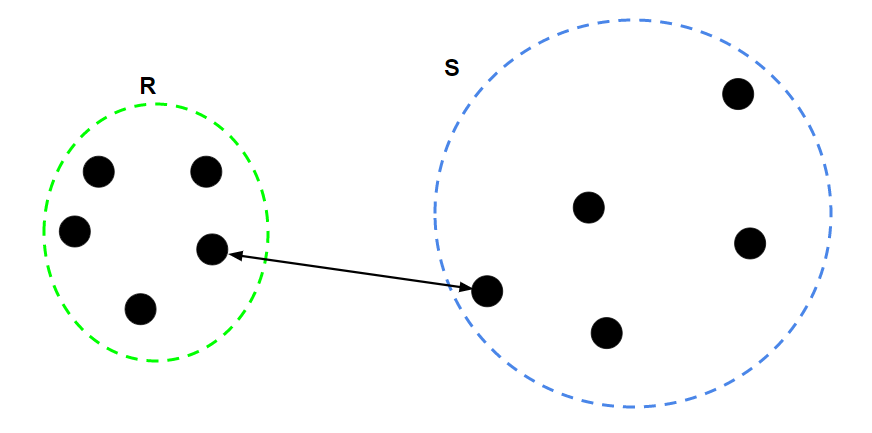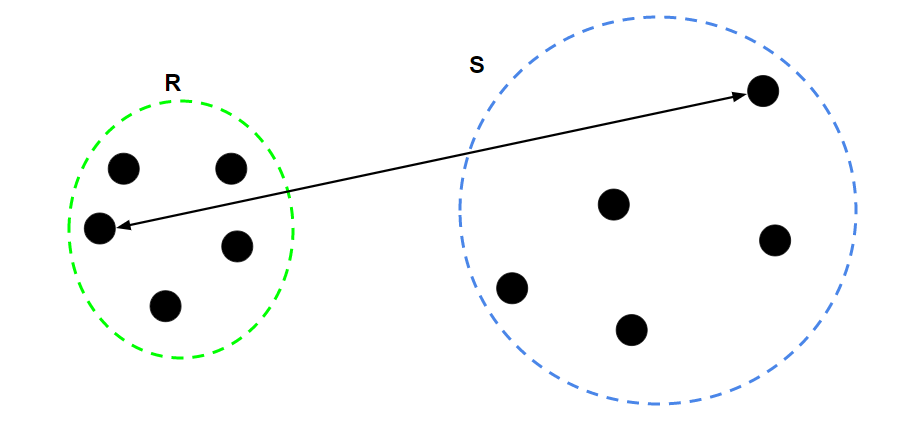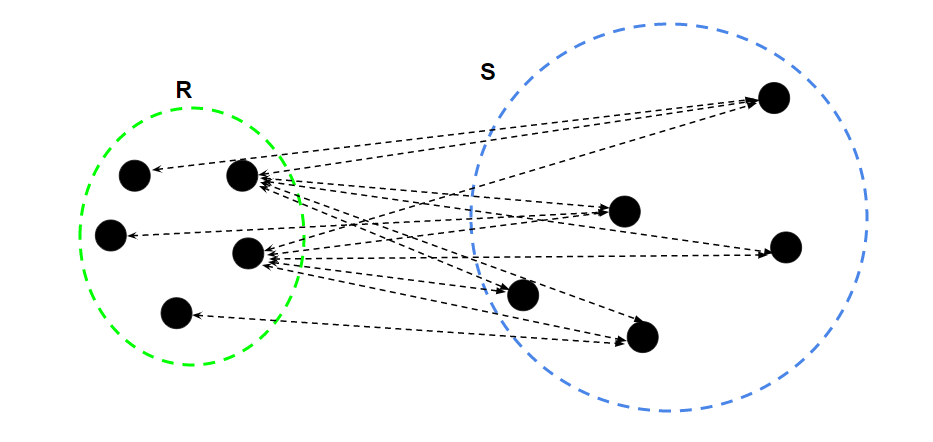# ML | Types of Linkages in Clustering

Prerequisites: Hierarchical Clustering

The process of Hierarchical Clustering involves either clustering sub-clusters(data points in the first iteration) into larger clusters in a bottom-up manner or dividing a larger cluster into smaller sub-clusters in a top-down manner. During both the types of hierarchical clustering, the distance between two sub-clusters needs to be computed. The different types of linkages describe the different approaches to measure the distance between two sub-clusters of data points. The different types of linkages are:-

1. Single Linkage: For two clusters R and S, the single linkage returns the minimum distance between two points i and j such that i belongs to R and j belongs to S.2. Complete Linkage: For two clusters R and S, the complete linkage returns the maximum distance between two points i and j such that i belongs to R and j belongs to S.3. Average Linkage: For two clusters R and S, first for the distance between any data-point i in R and any data-point j in S and then the arithmetic mean of these distances are calculated. Average Linkage returns this value of the arithmetic mean.where– Number of data-points in R– Number of data-points in S